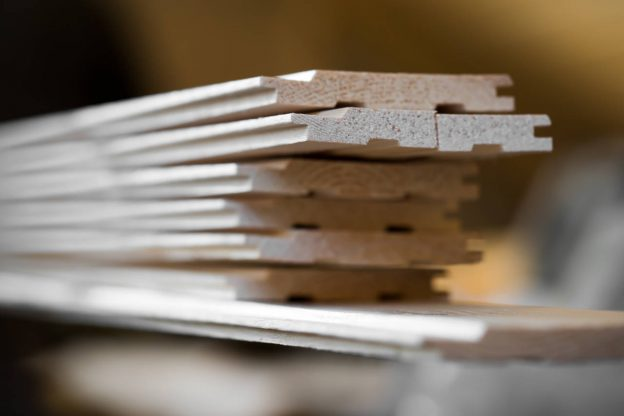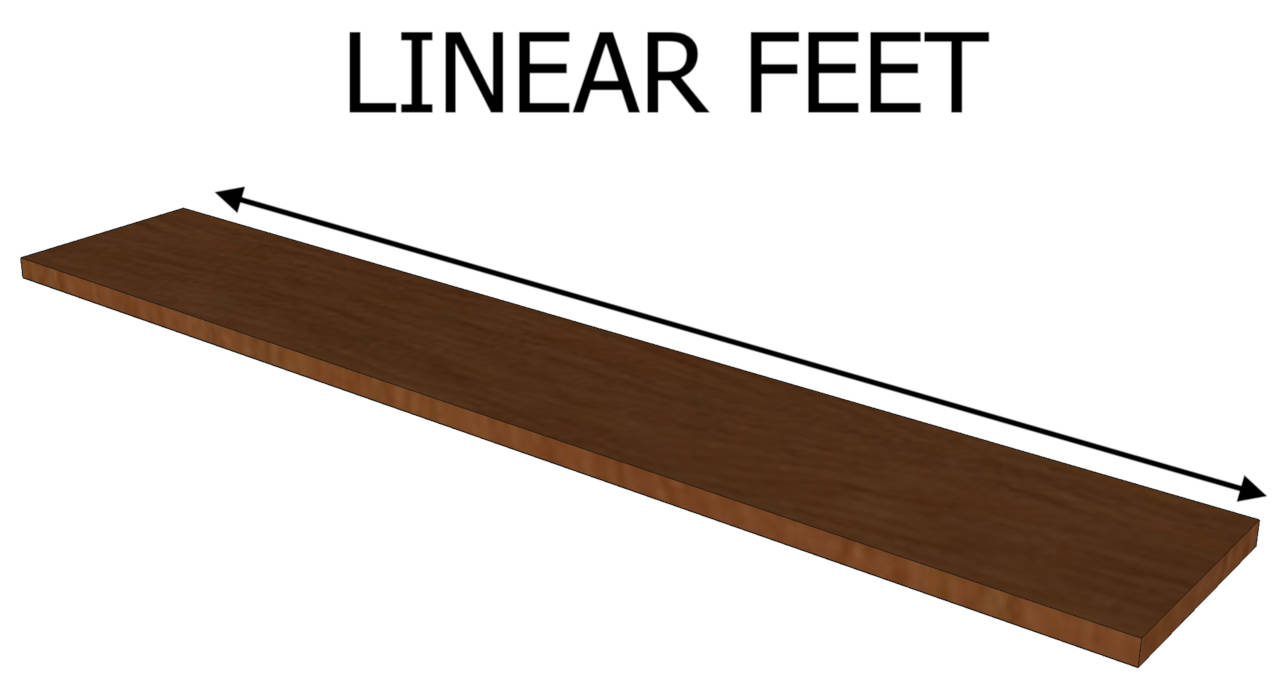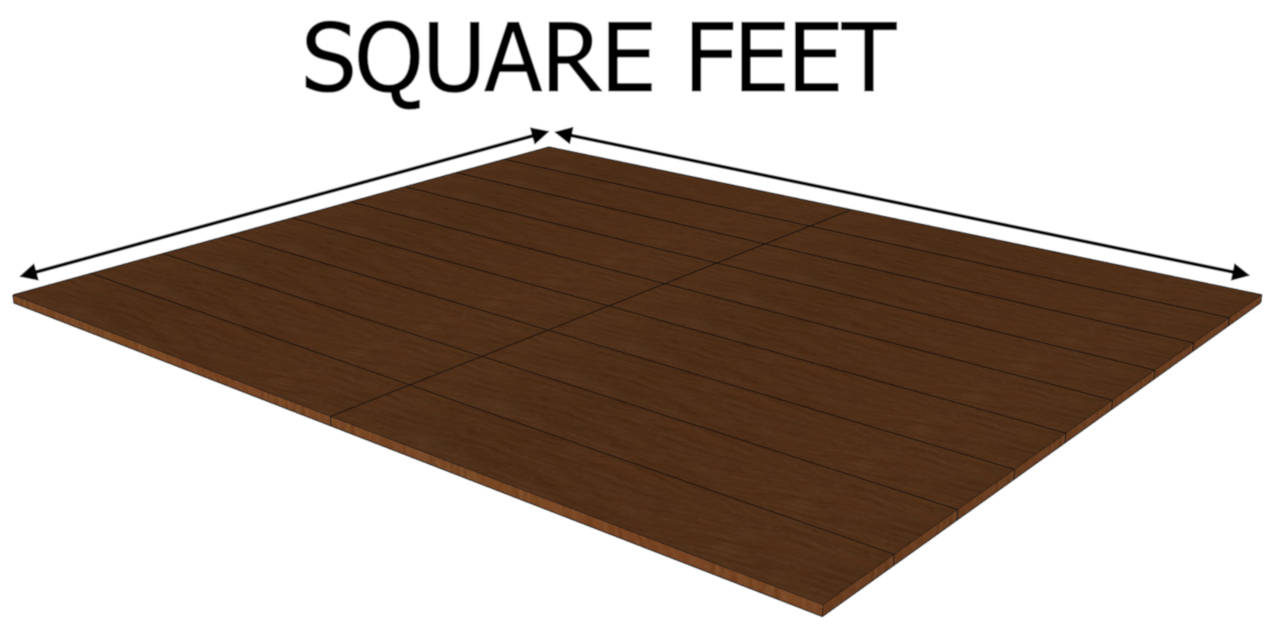# The Difference Between Linear Feet and Square FeetYou might see terms like linear foot and square foot used for construction materials like hardwood flooring or other types of lumber. These terms may refer to how a product is sold or installed, or they may refer to the size of the space you will be working with.

Prices for material or labor might be quoted by the square foot or linear foot, and it’s important to understand that these are very different forms of measurement.

You might be wondering what the difference is between the two.

## How Are Linear Feet and Square Feet Different?

Linear footage is a measurement of length or distance of material, while square footage is a measurement of the area of a space. These are different because linear footage measures the length of a line and the square footage of an area is a measure of a two-dimensional space.

For example, if you are installing crown molding around the ceilings of a room, you will need to know the number of linear feet of molding you will need. This means you need the total length that the new molding will travel.

But if you wanted to install tin ceiling tiles, you would need to know how big the ceiling is or how large the area is that you are covering – this is the square footage of the ceiling.

Some products like borders, countertop edging, lumber, and trim are sold by the linear foot measurement, since they are sold by length, rather than length and width.

For example, if you are installing new trim on your home, you only need the length of each area you will install it on. Likewise, if you are purchasing a new countertop and want a decorative edge, you need the total length of the countertop edge to determine how much the new edging would cost.

Products like flooring and services like painting are often sold or quoted by the square foot. Meaning that you need the total size of the area to get an accurate cost.

## How to Measure Linear Feet

Since linear feet measure the distance of a line, calculating linear feet can be as simple as measuring that distance. For example, to measure the linear feet of trim needed for a single wall, measure the wall from one end to the other.## How to Measure Square Feet

Measuring square feet is a little bit different because it’s a measure of area. To measure the square footage of a room, measure the length and width of the room, then multiply the two measurements together using a calculator.

The result is the total area of the space, or the spaces square footage. You can also use a square footage calculator for this task.## Can You Convert Square Feet to Linear Feet?

It’s possible to convert between linear feet and square feet, but you need some additional measurements. Because we’re switching between the distance of a single line and an area measurement, we need to know the width of the line as well as the length to convert from linear to square feet.

If, for example, you want to install a border inside around some flooring. The border will take up space, and you may wish to know how much square footage the border will take up from the total floor.

This way, you can subtract that amount from the rest of the flooring, potentially allowing you to order less of the regular flooring.

Start by finding the width of the border, then multiply that by the linear footage of the total length. Meaning that if you are installing 20′ of border and the border is 6″ wide, you would multiply 20′ × 0.5′ to get 10 square feet of border.

If the entire space is 120 square feet, you can order 110 square feet of standard flooring and your 20 linear feet of border and trust that you will fill the space.

Tools like our linear foot to square foot calculator make this process much easier.